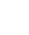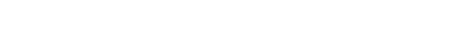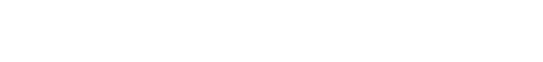## 3.SQUARES AND SQUARE ROOTS, CUBES AND CUBE ROOTS

Square:  Square number is the number raised to the power 2. The number obtained by the number multiplied by itself.

Ex: – 1) square of 5 = 52 = 5 × 5 = 25, 2) square of 3 = 32 = 3× 3 = 9

∗If a natural number p can be expressed as q2, where q is also natural, then p is called a square number.

Ex: – 1,4,9, …etc.

Test for a number to be a perfect square:

If a number is expressed as the product of pairs of equal factors, then it is called a perfect square.

Prime factors of 36 = 2× 2× 3× 3

36 can be expressed as the product of pairs of equal factors.

∴ 36 is a perfect square.

Square Root: the square root of a number x is that number when multiplied by itself gives x as the product. The square root of x is denoted byMethods of Finding Square root of given Number

Prime factorization method: –

Steps:

1. Resolve the given number into prime factors.
2. Make pairs of similar factors.
3. The product of prime factors, choosing one out of every pair gives the square root of the given number.

Ex: – To find the square root of 16

Prim factors of 16 = 2 ×2× 2× 2

= 2 × 2 = 4

∴ square root of 16 = 4

Division method: –

Steps:

1. Mark off the digits in pairs starting with the unit place. Each pair and remaining one digit are called a period.
2. Think of the largest number whose square is equal to or just less than the first period. Take this number as the divisor as well as quotient.
3. Subtract the product of divisor and quotient from the first period and bring down the next period to the right of the remainder. this becomes the new dividend.
4. Now, the new divisor is obtained by taking twice the quotient and annexing with it a suitable digit which is also taken as the next digit of the quotient, chosen in such a way that the product of the new divisor and this digit is equal to or just less than the new dividend.

Repeat steps 2, 3, and 4 till all the periods have been taken up. Thus, the obtained quotient is the required square root.

Ex: – To find the square root of 225Properties of a perfect square:

1. The square of an even number is always an even number.

Ex: – 22 = 4 (4 is even), 62 = 36 (36 is even), here 2, 6 are an even number.

2. The square of an odd number is always an odd number.

Ex: – 32 = 9 (9 is even), 152 = 225 (225 is even), here 3, 15 are an odd number.

3. The square of a proper fraction is a proper fraction less than the given fraction.

4. The square of decimal fraction less than 1 is smaller than the given decimal.

Ex: – (0.3)2 = 0.09 < 0.03.

5. A number ending with 2, 3, 7, or 8 is never a perfect square.

Ex: – 72, 58, 23 are not perfect squares.

6. A number ending with an odd no. of zeros is never a perfect square

Ex: – 20, 120,1000 and so on.

The square root of a number in decimal form

Make the no. of decimal places even, by affixing a zero, if necessary. Now periods and find out the square root by the long division method.

Put the decimal point in the square root as soon as the integral part is exhausted.

Ex: – To find the square root of 79.21The square root of a decimal number which is not perfect square:

if the square root is required to correct up to two places of decimal, we shall find it up 3 places of decimal and then round it off up to two decimal places.

if the square root is required to correct up to three places of decimal, we shall find it up 4 places of decimal and then round it off up to three decimal places.

Ex: – To find the square root of 0.8 up to two decimal placesCube of a number:

The cube of a number is that number raised to the power 3.

Ex: – cube of 0.3 = 0.33 = 0.027

Cube of 2 = 23 = 8

Perfect cube:

If a number is a perfect cube, then it can be written as the cube of some natural numbers.

Ex: – 1, 8, 27, and so on.

Cube root:

The cube root of a number x is that number which when multiplied by itself three times gives x as the product.Methods of finding the cube root of the given Number

Prime factorization method: –

Steps:

1. Resolve the given number into prime factors.
2. Make triplets of similar factors.
3. The product of prime factors, choosing one out of every triplet gives the cube root of the given number.

Ex: – 27

Prim factors of 27 = 3×3×3

= 3

∴ cube root of 27 = 3

Test for a number to be perfect cube:

A given number is a perfect cube if it can be expressed as the product of triplets of equal factors.

Prime factors of 2744 = 2×2×2 × 7×7×7

∴ 2744 is a perfect cube.

##### Visit my Youtube Channel: Click on Below Logo